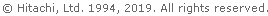HITACHI \$B%[!<%`(BComputer \$B!u(B Network System SiteGlobal Sites of related products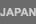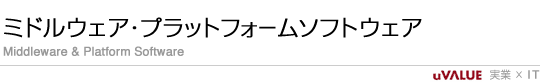|  \$B%[!<%`(B  |  \$B@=IJ!u%5!<%S%9(B  |  \$B%@%&%s%m!<%I(B  |  \$B;vNc>R2p(B  |  \$B%\$%Y%s%H!u%;%_%J!<(B  |\$BAm9g%5%\$%H%^%C%W(B\$BAm9g\$*Ld\$\$9g\$o\$;(B\$B8!:w(B by Google> \$B>\:Y\$J8!:w(B\$B%[!<%`(B > \$B\$?\$A\$^\$AK]Lu%Z!<%88x3+=*N;\$N\$*CN\$i\$;(B# \$B\$?\$A\$^\$AK]Lu%Z!<%88x3+=*N;\$N\$*CN\$i\$;(B\$B\$?\$A\$^\$AK]Lu%Z!<%8\$N8x3+\$O!\$(B2003\$BG/(B12\$B7n(B31\$BF|\$r\$b\$C\$F=*N;\$\$\$?\$7\$^\$7\$?!#(B
\$BK\@=IJ\$K4X\$9\$k%5!<%S%9\$d%5%]!<%H\$K\$D\$\$\$F\$O!\$(B\$BF|N)%*!<%W%s%_%I%k%&%'%"Ld\$\$9g\$o\$;%;%s%?!<(B\$B\$^\$G!\$\$*Ld\$\$9g\$o\$;\$/\$@\$5\$\$!#(B

\$B\$4MxMQ\$"\$j\$,\$H\$&\$4\$6\$\$\$^\$7\$?!#(B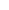\$B%H%T%C%/%9!u%K%e!<%9(B\$BG'Dj@)EY!u8&=\$(B\$B%Q!<%H%J!<%W%m%0%i%`(B\$B@=IJ%;%-%e%j%F%#(B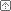\$B%Z!<%8%H%C%W\$X(B